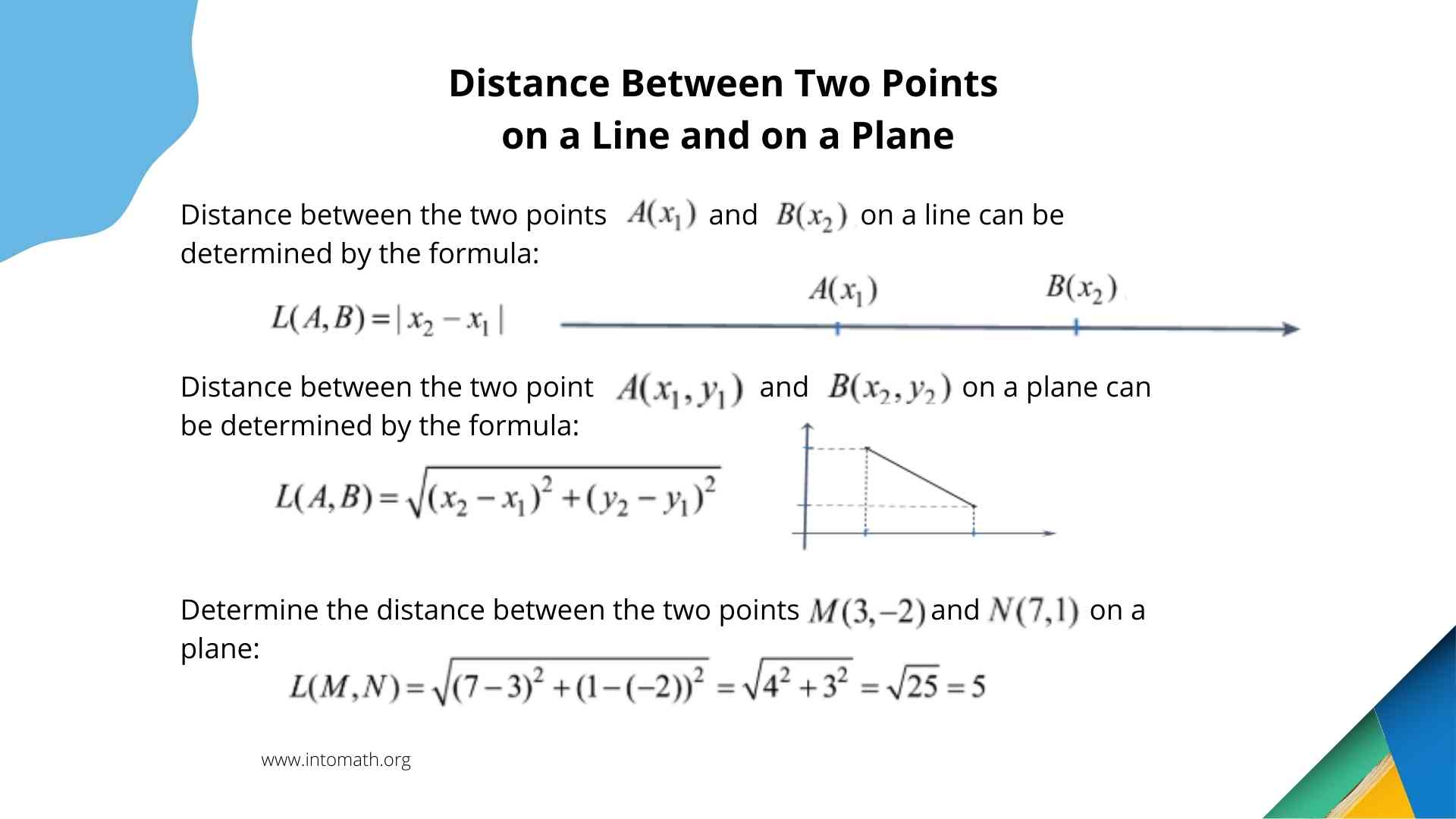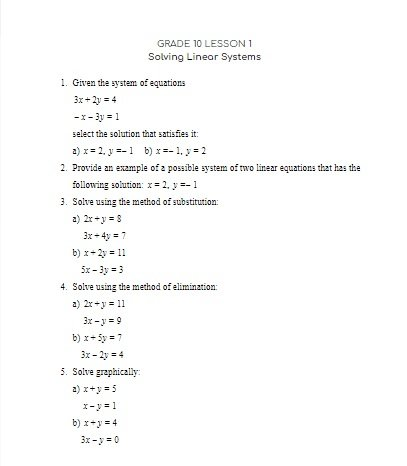CA$2.00 Coordinate geometry helps us find the distance between the points, determine the side lengths and angles of geometric shapes using a Coordinate Plane. There is a number of ways to do that and it is really fun to do when you understand why we take certain steps. In coordinate geometry you need to know some terminology. For example, you need to know that the word “origin” means a point with coordinates (0,0). Or that the word “subscript” means a small letter or number below a regular sized one (E-g.: x2). This lesson demonstrates how to determine the distance between the two points using their coordinates. It also explains how to find the midpoint on a line segment. In this lesson we are also looking at the equation of a circle. The general equation of a circle with the center not at the origin is (x – a)2 + (y – b)2 = r2 where r is the radius of a circle and a,b are not 0. When the center of a circle is at the origin, then a and b are equal to 0.Grade 10 Workbook (13 pages with answers) CA$4.00

In general, coordinate geometry is a fascinating branch of mathematics. It is like learning to read a mathematical map.

Did you know that any pdf file on the web is an example of a coordinate geometry? The words and symbols in these files are written using the coordinate geometry. If a document contains text and images, they are placed according to a Coordinate Plane using the concepts of a distance between two points, slope of a line, as well as trigonometry.

Photo editing software are based on coordinate geometry. Changing the dimensions of an image or moving it around the screen – everything is based on the concept and the position of every point has its coordinates (x,y).

In a GPS system that we use to get from one place to another, the longitude and the latitude of a place are its coordinates.